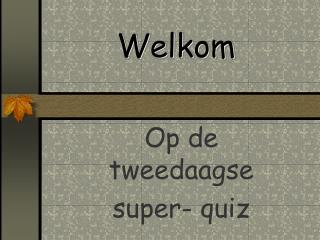DownloadDownload PresentationWelkom

# Welkom

Download Presentation## Welkom

- - - - - - - - - - - - - - - - - - - - - - - - - - - E N D - - - - - - - - - - - - - - - - - - - - - - - - - - -
##### Presentation Transcript

1. Welkom Op de tweedaagse super- quiz

2. Micro - macro

3. bv.

4. Oplossing

5. 1

6. 2

7. 3

8. 4

9. 5

10. Oplossingen

11. 1

12. 2

13. 3

14. 4

15. 5

16. Droedels

17. bv. ffffffff t zzzzzzz

18. Oplossing ffffffff t zzzzzzz = effe thee zetten

19. D K 1

20. G r 2

21. R ver ver 3 ver ver ver ver ver ver ver ver ver ver

22. 4 K L R

23. 5 M A Z T

24. Oplossingen

25. D K 1 = kind

26. G r 2 = ring

27. R ver ver 3 ver ver ver = revolver ver

28. 4 K L R = koploper

29. 5 M A Z T = emma zet thee

30. Micro - macro

31. 1

32. 2

33. 3

34. 4

35. 5

36. Oplossingen

37. 1

38. 2

39. 3

40. 4

41. 5

42. Piramides

43. bv. R A P T R A P P A T E R N P L A N T E R

44. Oplossing R A P T R A P P A T E R P A N T E R P L A N T E R

45. R E D R E D E N D R I E E N R E N D I E R 1

46. T E R S T E R A S T E R L A S T E R N 2 T E R

47. 3 S T E L S T E E L S T E L E N K L E T S E N A

48. D O E L D O L E N N L O N K E N D K L O O N D E N 4

49. R A A T S T R A A T T R A A G S T T R A A G S T E 5 R A A T T